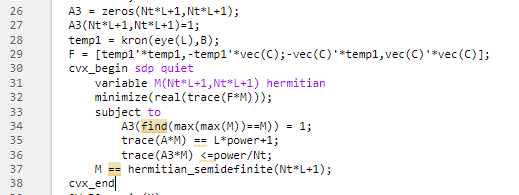# How variables defined in cvx are used for processingOne item in A3 needs to get the subscript that defines the largest element in variable M, and then make the element in A3 return the position of the subscript to 1, and then use it as a constraint

I’m not sure what you are trying to do. is it essentially
`max(M(:)) + M(Nt*L+1,Nt*L+1) <= power/Nt`, which is a convex constraint and would be accepted by CVX, presuming both sides evaluate to real.
Except that would need to be adjusted if the maximum element of `M` were the (NtL+1,NtL+1) element. And if the maximum element of `M` was off-diagonal, then there would be a (conjugate) transpose element as well. And what tf there’s a tie for maximum element (even aside from transpose element)? And of course, this doesn’t make sense if `M` is complex, which per your hernitian declaration it is.

I mean, when I affirm the constraints of row 38, I need to make further improvements to A3 by returning the row and column indexes corresponding to the elements with the largest absolute values in the set M matrix, and then setting the corresponding rows and columns in A3 to 1. Among them, 32 rows and 33 rows are the rows and columns corresponding to the elements that return the absolute value of the largest value in M, and the corresponding position in A3 is 1.

Of course, I also tried various rows and columns corresponding to the elements that returned the largest absolute value from the M matrix, and then set the corresponding rows and columns in A3 to 1, but there were problems, and I hope you can help me point out the problem. Rows 32 through 36 return the rows and columns corresponding to the absolute values of the largest elements in the M matrix and set the corresponding elements in A3 to 1.And the error is that there are too many output parameters

max of a CVX argument can’t do what max of a double precision argument does.

If something like `max(abs(M(:)))` doesn’t do what you want, you may need to use binary variables to model the max. See for instance, https://yalmip.github.io/tutorial/logicprogramming . However, there is no solver under CVX which can solve a Mixed-Integer SDP. So I think you would need to use YALMIP, with BNB as solver calling an SDP solver as uppersolver, or use cutsdp as solver…

help cvx/max

Disciplined convex/geometric programming information:
max is convex, log-log-convex, and nondecreasing in its first
two arguments. Thus when used in disciplined convex programs,
both arguments must be convex (or affine). In disciplined
geometric programs, both arguments must be log-convex/affine.

In the future, please copy and paste code into your post, and apply the Preformatted text icon, rather than posting an image. That way, forum readers can copy and paste your code into their own MATLAB session.

``````Nt = 9;
Nr = 9;
K = 4;
L = 20;
power = 10^(0/10);
fc = 10;
B = 10;
Tp = 2;
rou = 0.3;
H = (randn(Nt,K)+j*randn(Nt,K))/sqrt(2);
N_pbits = 2*K*L;
msg_bits = randi([0,1],1,N_pbits);
Y = reshape(QPSK_mapper_replace(msg_bits),[K,L]);%产生星座符号矩阵
X0 = chirp_generator( Nt,fc,Tp,B );
p1 = sqrt(rou);%根号rou
p2 = sqrt(1-rou);
at = [1;exp(-j*pi/2);exp(-j*pi);exp(-j*pi*3/2);exp(-j*pi*2);exp(-j*pi*5/2);exp(-j*pi*3);exp(-j*pi*7/2);exp(-j*pi*4)]';
ar = [0,-j*pi*at(2)*sqrt(3)/2,-j*pi*at(3)*sqrt(3),-j*pi*at(4)*3/2*sqrt(3),-j*pi*at(5)*2*sqrt(3),-j*pi*at(6)*5/2*sqrt(3),-j*pi*at(7)*3*sqrt(3),-j*pi*at(8)*7/2*sqrt(3),-j*pi*at(9)*4*sqrt(3)].';
s = j*sqrt((1-rou)*1/3);
aj = ar*at;
B = [p1*H,s*aj].';%eye函数表示对角元素为1，其余全为零的N*N矩阵;.'表示一般转置
C = [p1*sqrt(power)*Y;zeros(Nr,L)];%根号power*Y=S的转置，X_arbi等于参考波形X0的转置
A1 = diag([ones(1,Nt*L),0]);
A2(Nt*L+1,Nt*L+1) = 1;
A = A1+A2;
temp1 = kron(eye(L),B);
F = [temp1'*temp1,-temp1'*vec(C);-vec(C)'*temp1,vec(C)'*vec(C)];
A3=zeros(Nt*L+1,Nt*L+1);
A3(Nt*L+1,Nt*L+1)=1;
cvx_begin sdp
variable M(Nt*L+1,Nt*L+1) hermitian
[m,im] = (max(abs(M)));
[m2,im2]=max(m);
a = im(im2);
b = im2;
A3(a,b) = 1;
minimize(real(trace(F*M)));
subject to
M(Nt*L+1,Nt*L+1)==1;
trace(A*M) == L*power+1;
trace(A3*M)<=power/Nt+1;
M == hermitian_semidefinite(Nt*L+1);
cvx_end
[V,D] = eig(M);
[value,num] = max(diag(D));
x = sqrt(value)*V(:,num);
X = reshape(x(1:Nt*L),Nt,L);
``````

According to you, you can’t use the max function in CVX, then I am in my code, because I should put the row and column parts corresponding to the maximum value of my matrix M, that is: [m, im] = (max(abs(M)));
[m2,im2]=max(m);
a = im(im2);
b = im2;
A3(a,b) = 1;

max applied to a CVX argument returns just the maximum, not also the index. if you want the index, you will have to do something along the lines of the YALMIP link in my previous post.

``````Nt = 9;
Nr = 9;
K = 4;
L = 20;
power = 10^(0/10);
fc = 10;
B = 10;
Tp = 2;
rou = 0.3;
H = (randn(Nt,K)+j*randn(Nt,K))/sqrt(2);
N_pbits = 2*K*L;
msg_bits = randi([0,1],1,N_pbits);
Y = reshape(QPSK_mapper_replace(msg_bits),[K,L]);%产生星座符号矩阵
X0 = chirp_generator( Nt,fc,Tp,B );
p1 = sqrt(rou);%根号rou
p2 = sqrt(1-rou);
at = [1;exp(-j*pi/2);exp(-j*pi);exp(-j*pi*3/2);exp(-j*pi*2);exp(-j*pi*5/2);exp(-j*pi*3);exp(-j*pi*7/2);exp(-j*pi*4)]';
ar = [0,-j*pi*at(2)*sqrt(3)/2,-j*pi*at(3)*sqrt(3),-j*pi*at(4)*3/2*sqrt(3),-j*pi*at(5)*2*sqrt(3),-j*pi*at(6)*5/2*sqrt(3),-j*pi*at(7)*3*sqrt(3),-j*pi*at(8)*7/2*sqrt(3),-j*pi*at(9)*4*sqrt(3)].';
s = j*sqrt((1-rou)*1/3);
aj = ar*at;
B = [p1*H,s*aj].';%eye函数表示对角元素为1，其余全为零的N*N矩阵;.'表示一般转置
C = [p1*sqrt(power)*Y;zeros(Nr,L)];%根号power*Y=S的转置，X_arbi等于参考波形X0的转置
A1 = diag([ones(1,Nt*L),0]);
A2(Nt*L+1,Nt*L+1) = 1;
A3(1,1) = 1;
A = A1+A2;
temp1 = kron(eye(L),B);
F = [temp1'*temp1,-temp1'*vec(C);-vec(C)'*temp1,vec(C)'*vec(C)];
A3=zeros(Nt*L+1,Nt*L+1);
A3(Nt*L+1,Nt*L+1) = 1;
A3(1,1) = 1;
cvx_begin sdp
variable M(Nt*L+1,Nt*L+1) hermitian
M(Nt*L+1,Nt*L+1)=0;
M(1,1) = max(max(M));
minimize(real(trace(F*M)));
subject to
M(Nt*L+1,Nt*L+1)==1;
trace(A*M) == L*power+1;
trace(A3*M)<=power/Nt+1;
M == hermitian_semidefinite(Nt*L+1);
cvx_end
[V,D] = eig(M);
[value,num] = max(diag(D));
x = sqrt(value)*V(:,num);
X = reshape(x(1:Nt*L),Nt,L);
``````

As you said, it is possible to use the max function in cvx, but why did I use it or report an error.

`M(1,1) = max(max(M));` It is a nonlinear constraint. It is non-convex. You may have to resort to use of binary variables, as per my previous post and link, to do what you want.